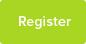# E=mc² - A Note On Units

A follow up to my last article in which I explain the most common units used in mass-energy calculations

Date : 24/11/2018### Author Information

Subject : Physics

In my last post, I talked about some of the problems A Level Physics students encounter in mass-energy (E=mc2) calculations. In passing, I mentioned use of unfamiliar units during the calculations. This is a guide to some of the most common units used in the calculations.

Mass-energy equivalence calculations use the iconic equation E=mc2. The equation E=mc² is often written as

ΔE = c²Δm

Where:

ΔE = Energy released in Joules (J)

Δm = Mass defect (Decrease in TOTAL particle ) in kilograms (kg)

c² = a constant equal to the speed of light squared (≈ 3 x 1016)

Joules and kilograms are the SI units for energy and mass, respectively. However, A Level Physics students familiar with mass-defect questions will have noticed that these questions rarely use joules or kilograms as units. That’s because the energy released and the mass defect in a single nuclear event (decay, fission, fusion) are very, very small quantities which involves tiny numbers if calculated in joules or kilograms.

More often, A Level Physics questions on mass-defect and binding energy use Atomic Mass Units (a.m.u. or just u for short) for mass and MeV (mega-electronvolts) for energy. Atomic mass units are, as the name suggests, the units used for the atomic mass of atomic nuclei, where protons and neutrons each have a mass of approximately 1u. For mass-energy calculations, very precise values for mass are used: often 5 or 6 significant figures (s.f.), precise enough to record different values for protons and neutrons. The “conversion factor” between a.m.u. and MeV is not c2 but 931.5. That is to say, 1u is roughly equivalent to 931.5MeV.

The other set of units student may encounter are MeV for energy and MeV/c2 for mass. This is simply a set of units which incorporates the constant c2 into the unit for mass itself so that the numbers for equivalent mass and energy are the same. E.g. an electron which has a rest energy of 0.511 MeV will have a rest mass of 0.511 MeV/c2 . Think of filling a car with petrol: we almost never pump a particular volume of petrol measured in litres or gallons. Instead, you pump £20 worth of petrol or £30 or £50. The volume of petrol which we have pumped has been measured in terms of it’s equivalent cost in pounds. So if we ask “what is the cost of £50 worth of petrol?” the answer is obvious: £50 because we pumped that particular volume in order that it cost exactly £50. Similarly, if the rest mass of an electron is 0.511 MeV/ c2 its rest energy will be 0.511 MeV since the mass has been calculated here according to the equivalent rest energy.

In my last post, I talked about some of the problems A Level Physics students encounter in mass-energy (E=mc2) calculations. In passing, I mentioned use of unfamiliar units during the calculations. This is a guide to some of the most common units used in the calculations.

Mass-energy equivalence calculations use the iconic equation E=mc2. The equation E=mc² is often written as

ΔE = c²Δm

Where:

ΔE = Energy released in Joules (J)

Δm = Mass defect (Decrease in TOTAL particle ) in kilograms (kg)

c² = a constant equal to the speed of light squared (≈ 3 x 1016)

Joules and kilograms are the SI units for energy and mass, respectively. However, A Level Physics students familiar with mass-defect questions will have noticed that these questions rarely use joules or kilograms as units. That’s because the energy released and the mass defect in a single nuclear event (decay, fission, fusion) are very, very small quantities which involves tiny numbers if calculated in joules or kilograms.

More often, A Level Physics questions on mass-defect and binding energy use Atomic Mass Units (a.m.u. or just u for short) for mass and MeV (mega-electronvolts) for energy. Atomic mass units are, as the name suggests, the units used for the atomic mass of atomic nuclei, where protons and neutrons each have a mass of approximately 1u. For mass-energy calculations, very precise values for mass are used: often 5 or 6 significant figures (s.f.), precise enough to record different values for protons and neutrons. The “conversion factor” between a.m.u. and MeV is not c2 but 931.5. That is to say, 1u is roughly equivalent to 931.5MeV.

The other set of units student may encounter are MeV for energy and MeV/c2 for mass. This is simply a set of units which incorporates the constant c2 into the unit for mass itself so that the numbers for equivalent mass and energy are the same. E.g. an electron which has a rest energy of 0.511 MeV will have a rest mass of 0.511 MeV/c2 . Think of filling a car with petrol: we almost never pump a particular volume of petrol measured in litres or gallons. Instead, you pump £20 worth of petrol or £30 or £50. The volume of petrol which we have pumped has been measured in terms of it’s equivalent cost in pounds. So if we ask “what is the cost of £50 worth of petrol?” the answer is obvious: £50 because we pumped that particular volume in order that it cost exactly £50. Similarly, if the rest mass of an electron is 0.511 MeV/ c2 its rest energy will be 0.511 MeV since the mass has been calculated here according to the equivalent rest energy.

This resource was uploaded by: Thomas